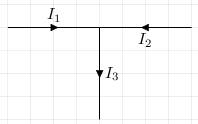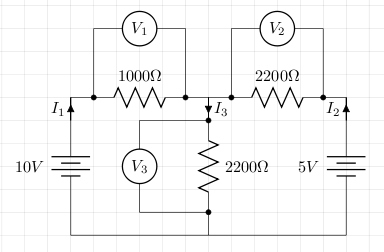# Kirchhoff's Laws

There are two Kirchhoff's laws: one for current, and one for voltage.

## Current Law

The current law states that the sum of the currents entering a node is equal to the sum of the currents leaving a node. In the circuit below, I3 = I1 + I2.## Voltage Law

The voltage law states that in any loop, the sum of the voltage drops is equal to the sum of the EMFs. The circuit below shows three loops, two EMFs (the two batteries), and three voltage drops (the resistors). In the first loop we have 10 = V1 + V3, in the second we have 5 = V2 + V3, and in the third we have 5 = V2 - V1 + 10 (or alternatively 10 = V1 - V2 +5).## Example

With the circuit above we can use both of Kirchhoff's laws to calculate the three currents (I1, I2, and I3) and the three voltage drops (V1, V2, and V3). We do this by solving simultaneous equations with the matrix method.

First we write down our equations. In this example they are as follows:

• I1 + I2 - I3 = 0 (Kirchhoff's current law)
• 0*I1 + 2200*I2 + 2200*I3 = 5 (Kirchhoff's voltage law)
• 1000*I1 + 0*I2 + 2200*I3 = 10 (Kirchhoff's voltage law)

We take the coefficients of these equations and construct our initial matrix:

 1 1 -1 0 0 2200 2200 5 1000 0 2200 10

We then follow the usual matrix method (or use a calculator or computer). The result derived manually was:

 1 0 0 0.00372 0 1 0 -0.0008 0 0 1 0.00292

So this gives us I1 = 3.72mA, I2 = -0.8mA and I3 = 2.92mA. From this we can now calculate V1 = 3.6V, V2 = -1.54V, and V3 = 6.38V.

The circuit was built and measurements were taken to compare theory and practice. The result is shown in the table below.

I1 I2 I3 V1 V2 V3 0.0036 -0.0006 0.003 3.55 -1.43 6.48 0.00372 -0.0008 0.00292 3.6 -1.54 6.38

## References

Fischer-Cripps. A.C., The Electronics Companion. Institute of Physics, 2005.